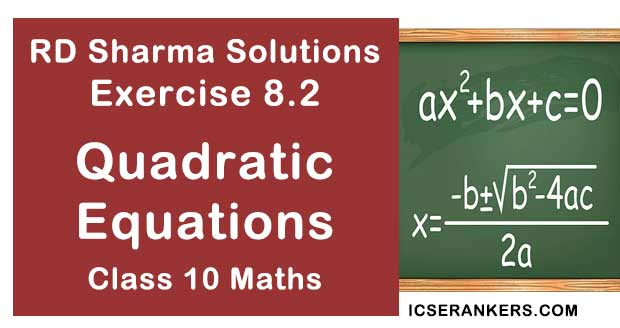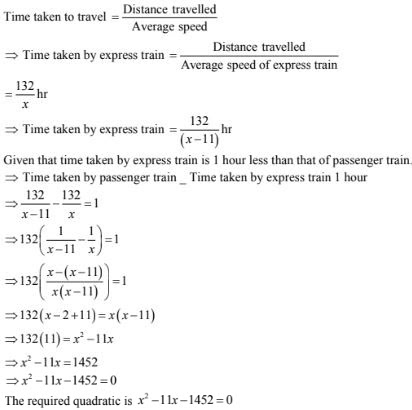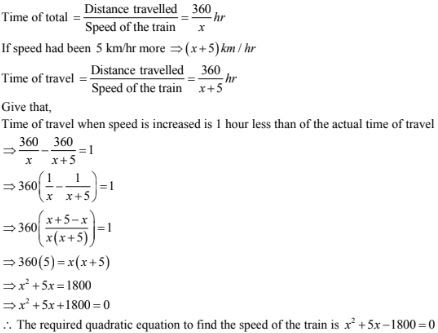# RD Sharma Solutions Chapter 8 Quadratic Equations Exercise 8.2 Class 10 MathsChapter Name RD Sharma Chapter 8 Quadratic Equations Book Name RD Sharma Mathematics for Class 10 Other Exercises Exercise 8.1Exercise 8.3Exercise 8.4Exercise 8.5Exercise 8.6 Related Study NCERT Solutions for Class 10 Maths

### Exercise 8.2 Solutions

1. The product of two consecutive positive integers is 306. Form the quadratic equation to find the integers, if x denotes the smaller integer.

Solution

Given that the smallest integer of 2 consecutive integer is denoted by x
⇒ The two integer will be x and (x + 1)
Product of two integers ⇒ x(x + 1)
Given that the product is 306
∴ x(x+ 1) = 306
⇒ x2 + x = 306

⇒ x2 + x - 306 = 0
∴ The required quadratic equation is x2 + x - 306 = 0

2. John and Jivanti together have 45 marbles. Both of them lost 5 marbles each, and the product of the number of marbles they now have is 128. Form the quadratic equation to find how many marbles they had to start with, if John had x marbles.

Solution

Given that John and Jivani together have 45 marbles and John has x marbles
⇒ Jivani had (45 - x ) marbles
No. of marbles John had after loosing 5 marbles = x - 5
No. of marbles Jivani had after loosing 5 marbles = (45 - x) - 5
= 45 - 5 -x
= 40 - x
Given that product of the no. of marbles they now have = 128
⇒ (x - 5)(40 - x) = 128
⇒ 40x - x2 - 40 ×5 + 5x = 128
⇒ 45x - x2  - 200 = 128
⇒ x2 - 45x + 128 + 200 = 0
⇒ x2 - 45x + 328 = 0
∴ The required quadratic equation is x2 - 45x + 328 = 0

3. A cottage industry produces a certain number of toys in a day. The cost of production of each toy(in rupees) was found to be 55 minus the number of articles produced in a day. On a particular day, the total cost of production was Rs. 750. If x denotes the number of toys produced that day, form the quadratic equation to find x.

Solution

Given that x denotes the no of toys product in a day
⇒ The cost of production of each by = 55 - no. of toys produced in a day = (55 - x)
Total cost of production is nothing but product of no. of toys produced in a day and cost of production of each toy
⇒ x(55 -x)
But total cost of production  = Rs 750
⇒ x(55 -x )
But total cost of production = Rs 750
⇒ x(55 - x) = 750
⇒55x - x2 = 750
⇒ x2 - 55x + 750 = 0
∴ The required quadratic from of the given data is x2 - 55x + 750 = 0

4. The height of a right triangle is 7 cm less than its base. If the hypotenuse is 13 cm, form the quadratic equation to find the base of the triangle.

Solution

Given that in a right triangle is 7 cm less than its base
Let base of the triangle be denoted by x
⇒ Height of the triangle = (x - 7) cm
We have hypotenuse of the triangle
(base)2 + (height)2 = (Hypotenuse)2
⇒ (x)2 + (x - 2)2 = (13)2
⇒ x2 + x2 - 14x + 49 = 169
⇒ 2x2 - 14x + 49 - 169 = 0
2x2 - 14x - 120 = 0
⇒ 2(x2 - 7x - 60) = 0
⇒ x2 - 7x - 60 = 0
∴ The required quadratic equation is x2 - 7x -60 = 0

5. An express train takes 1 hour less than a passenger train to travel 132 km between Mysore and Bangalore. If the average speed of the express train is 11 km/hr more than that of the passenger train , form the quadratic equation to find the average speed of express train.

Solution

Let the arrange speed of express train be denoted by x km/hr
Given that average speed of express train is 11 km/hr more than that of the passenger train
⇒ Average speed of passenger train = x = 11 km/hr
Total distance travelled by the train  = 132 km
We know that,
Time taken to travel = (Distance travelled)/Average speed)6. A train travels 360 km at a uniform speed. If the speed had been 5km/hr more, it would have taken 1 hour less for the same journey. Form the quadratic equation to find the speed of the train.

Solution

Let speed of train be x km/hr
Distance travelled by trin = 360 km
We know that